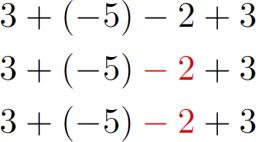# Variables: 3140

Determine the value of the expression 3a + 2b - a2 - 4b2 for the values ​​of the variables:
a) a = - 1, b = 3
b) a = 2, b = -1
c) a = -2, b = -3
d) a = 4, b = 2
e) a = -5, b = 0

A =  -34
B =  -4
C =  -52
D =  -16
E =  -40

### Step-by-step explanation:Did you find an error or inaccuracy? Feel free to write us. Thank you!